# Pro-p group

(diff) ← Older revision | Latest revision (diff) | Newer revision → (diff)

A profinite group that is a projective limit of finite-groups (cf.-group). E.g. the additive group of the ringof-adic integers is a pro--group. In Galois theory pro--groups appear as Galois groups of-extensions of fields.

Letbe a pro--group. A system of generators ofis a subset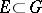with the properties: 1)is the smallest closed subgroup ofgenerated by; and 2) any neighbourhood of the identity ofcontains almost-all (i.e. all except a finite number of) elements of.

Letbe an index set and let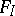be the abstract free group with system of generators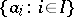. The projective limit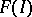of the system of groups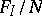, whereis a normal subgroup ofsuch that the index ofinis a power of a number, while almost-all elements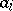,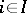, lie in, is a pro--group, called the free pro--group with system of generators. Every closed subgroup of a free pro--group is itself a free pro--group. Any pro--groupis a quotient group of a free pro--group, i.e. there is an exact sequence of homomorphisms of pro--groups,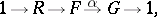whereis a suitable free pro--group. (This sequence is called a presentation ofby means of.) A subsetis called a system of relations ofifis the smallest closed normal subgroup incontainingand if every open normal subgroup incontains almost-all elements of. The cardinalities of a minimal (with respect to inclusion) set of generators and a minimal system of relations of a corresponding presentation of a pro--grouphave a cohomological interpretation: The first cardinality is the dimension over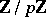of the space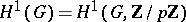, while the second is the dimension overof the space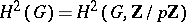. Hereis regarded as a discrete-module with a trivial-action. Ifis a finite-group, then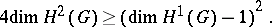This result implies a negative solution to the classical class field tower problem (cf. Tower of fields) .

How to Cite This Entry:
Pro-p group. Encyclopedia of Mathematics. URL: http://encyclopediaofmath.org/index.php?title=Pro-p_group&oldid=15018
This article was adapted from an original article by V.L. Popov (originator), which appeared in Encyclopedia of Mathematics - ISBN 1402006098. See original article